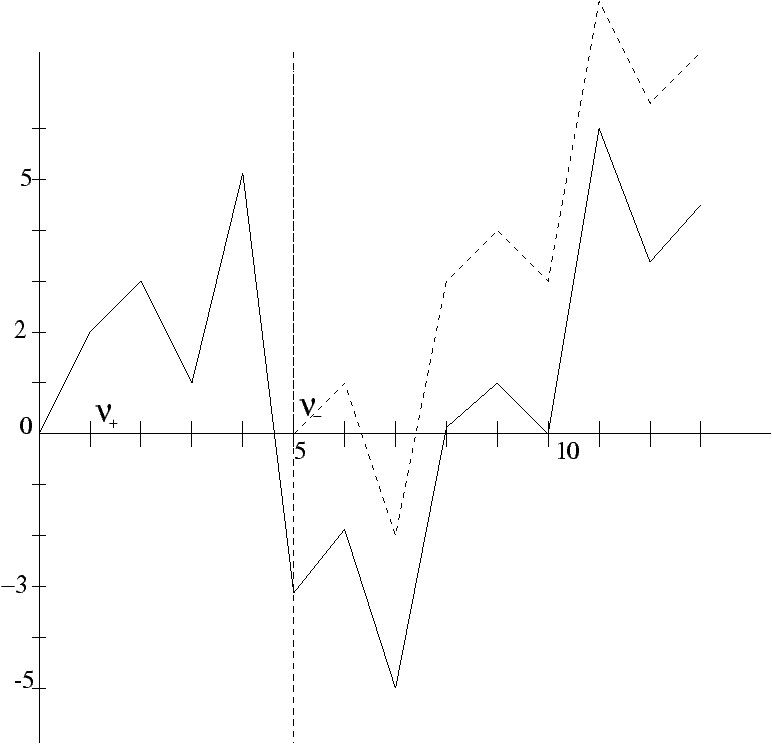Wiener-Hopf Factorization: Probabilistic Methods

Philippe Robert

Inria Rocquencourt

Algorithms Seminar

March 17, 1997

[summary by Jean-François Dantzer]

A properly typeset version of this document is available in postscript and in pdf.

If some fonts do not look right on your screen, this might be fixed by configuring your browser (see the documentation here).

## 1   Introduction

We consider a discrete random walk on Z, defined by
S0=0    and      Sn=
 n å i=1
Xi,   n>0,
where (Xi)i³ 1 is an independent identically distributed (i.i.d.) sequence of random variables. We define two hitting times n+ and n-,
n+=inf{k>0/Sk>0},    n-=inf{k>0/Sk£ 0},
with the convention inf(Ø)=+¥.Figure 1: Random walks (Sn)n³ 0 and (Sn+n-)n³ 0 (in dotted line)

We also define M and L two variables indicating respectively the maximum of the random walk and the hit moments at which it is attained
M=
 sup n³ 0
{Sn},     L=
 inf n³ 0
{Sn=M}.
This talk presents the classical probabilistic methods to derive the join distributions of (n+,Sn+), (n-,Sn-) and (M,L).

Applications of these results have been found in biology [3, 4] or in queueing theory . For more details on this subject, see [1, 5].

## 2   Distribution of (n+,Sn+) and (n-,Sn-)

The distributions of the pairs (n+,Sn+) and (n-,Sn-) will be expressed through their generating functions, i.e., E(un+zSn+) and E(un-zSn-).

We consider three variables
Y+(u,z)
=
1
1-E(u
 n+
z
S
 n+

)

on     {|u|<1,|z|£ 1},
Y-(u,z)
=
1
1-E(u
 n-
z
S
 n-

)

on     {|u|<1,|z|³ 1},
Y (u,z)
=
 å n³ 0
E(unz
 Sn
)
on     {|u|<1,|z|= 1}.
The main result, the factorization of Wiener-Hopf described in the following proposition, gives an analytic characterization of Y+ and Y-.
Proposition 1

Y can be uniquely decomposed on {|z|=1} as:
Y (u,z)=Y+ (u,z)Y- (u,z),
where Y+ and Y- have the following properties:
• Y+ is analytic on {|z|<1};
• Y+ and 1/Y+ are continuous and bounded on {|z|£ 1};
• Y+(u,0)=1;
and
• Y- is analytic on {|z|>1};
• Y- and 1/Y- are continuous and bounded on {|z|³ 1}.

Proof. The proof is based on the following arguments:
• The function Y can be expressed with the distribution of X1, the sequence (Xi)n³ 1 is independent identically distributed, then E(ZSn)=E(Zåi=1nXi)=E(ZX1)n, thus
Y (u,z)=  å n³ 0
un E(z  X1
)n=
1
1-uE(z  X1
)
;
• (Sn)n³ 0 and (Sn+n-)n³ 0 have same distribution, (Sn+n-)n³ 0 is the random walk beginning at the time n-;
• The independence between the pair (n-,Sn-) and the sequence (Sn+n-)n³ 0;
• the same properties are also valid for (Sn+n+)n³ 0.

## 3   Examples

The factorization is easy when Y has a finite number of poles and zeros. We consider two such examples.

### 3.1   Random walk ± 1

We suppose Xi=1 with probability p and Xi=-1 with probability (1-p). In that case,
Y(u,z)=
z
-upz2+z-u(1-p)
Y has only two poles
a1(u)=
1-(1-4u2p(1-p))1/2
2up
,    a2(u)=
1+(1-4u2p(1-p))1/2
2up
,
with     0£a1(u)£ 1£a2(u).

The decomposition gives:
Y+(u,z)=
a2(u)
a2(u)-z
and     Y-(u,z)=
z
a2(u)up(z-a1(u))
.

Here, we obtain the generating functions:
E(u
 n+
z
S
 n+

)=
z
a2(u)
,     E(u
 n-
z
S
 n-

)=(1-a2(u)up)+
u(1-p)
z
.

### 3.2   Random walk left bounded

We suppose Pr(Xi<-1)=0.
Y(u,z)=
1
1-uE(zX)
=
z
z-uE(zX+1)
.
In that case, the factorization is easy because by Rouché's theorem the function z|® z-uE(zX+1) has one only root which belongs to {|z|<1}, which we denote by a (u). One proves that a (u)Î [0,1] and the decomposition of Y is the following:
Y+(u,z)=
z-a (u)
z-uE(zX+1)
uPr(X=-1)
a (u)
,     Y-(u,z)=
z
z-a (u)
a (u)
Pr(X=-1)
,
and the generating functions are:
E(u
 n-
z
S
 n-

)
=1-
uPr(X=-1)
a (u)
+
uPr(X=-1)
z
,
E(u
 n+
z
S
 n+

)
=1-
a (u)
uPr(X=-1)
+
z-uE(zX+1)
z-a (u)
.

## 4   Distribution of the Maximum and its first Hitting time (M,L)

The distribution of the pair (M,L) is expressed through its generating function E(xLzM).

Proposition 2

E(xLzM)=
 lim u® 1
Y+(ux,z)
Y+(u,1)
.

Proof. We define the variables Mn and Ln, as
Mn=
 max 0£ k£ n
Sk,     Ln=inf{k/Sk=Mn},
and the function H on {|u|<1,|x|< 1, |z|< 1} as
H(u,x,z)=E(
 å n³ 0
unx
 Ln
z
 Mn
).

Using the same arguments as in proposition 1, it can be proved that:
H(u,x,z)=
Y+(ux,z)
Y+(u,1)(1-u)
.

We conclude applying
 lim u® 1
(1-u)H(u,x,z)=
 lim u® 1
(1-u)E(
 å n³ 0
unx
 Ln
z
 Mn
)=
 lim n® +¥
E(x
 Ln
z
 Mn
)=E(xLzM).

For the case of the random walk of subsection 3.1, it gives for p<1/2:
E(xLzM)=
a2(u)
a2(u)-z
1-2p
1-p
,
then
Pr(L<+¥,M<+¥)=1,
and the distribution of M is geometric with parameter p/1-p, for p>1/2:
E(xLzM)=0     and     Pr(L=M=+¥)=1.

## References


Feller (William). -- An introduction to probability theory and its applications. -- John Wiley & Sons, New York, 1971, 2nd edition, vol. II.


Iglehart (Donald L.). -- Extreme values in the GI/G/1 queue. Annals of Mathematical Statistics, vol. 43, n°2, 1972, pp. 627--635.


Karlin (Samuel) and Altschul (Stephen F.). -- Methods for assesing the statistical significance of molecular sequences features by using general scoring schemes. Proceedings of the National Academy of Sciences of the USA, vol. 87, 1990, pp. 2264--2268.


Karlin (Samuel) and Dembo (Amir). -- Strong limit theorems of empirical functionals for large exedances of partial sums of i.i.d. variables. Annals of Probability, vol. 19, n°4, 1991, pp. 1737--1755.


Spitzer (F.). -- Principles of Random Walk. -- Van Nostrand, 1964.

This document was translated from LATEX by HEVEA.